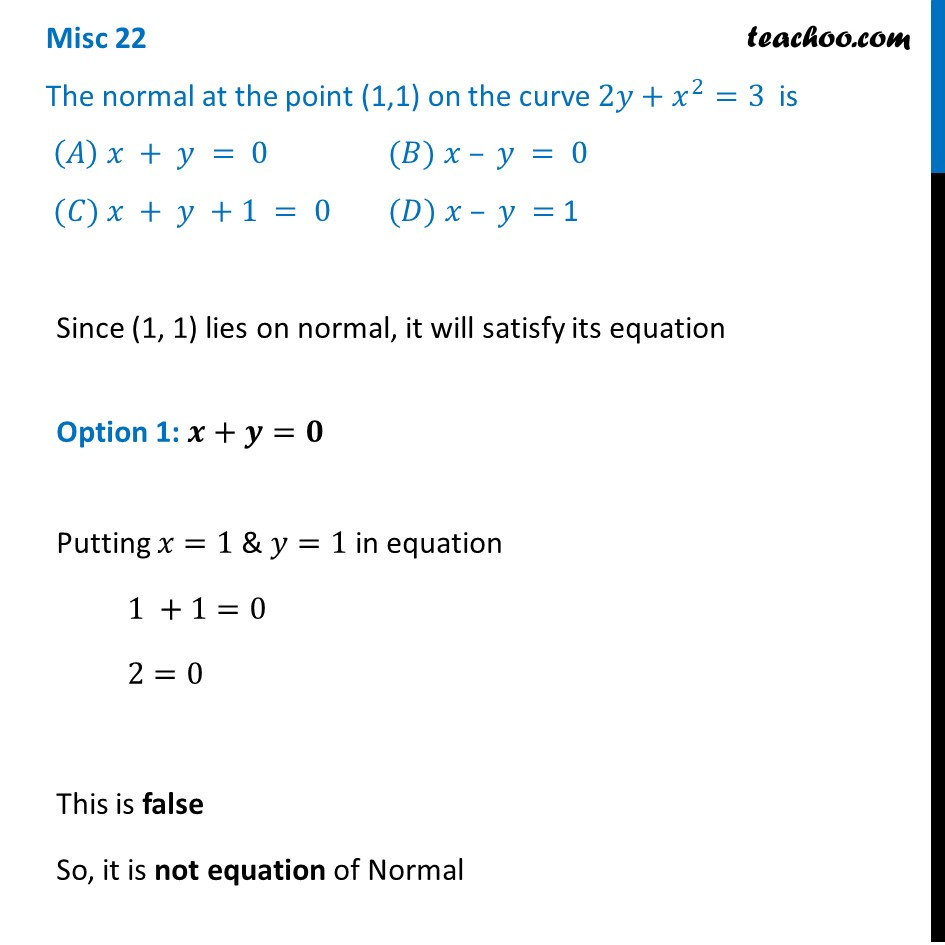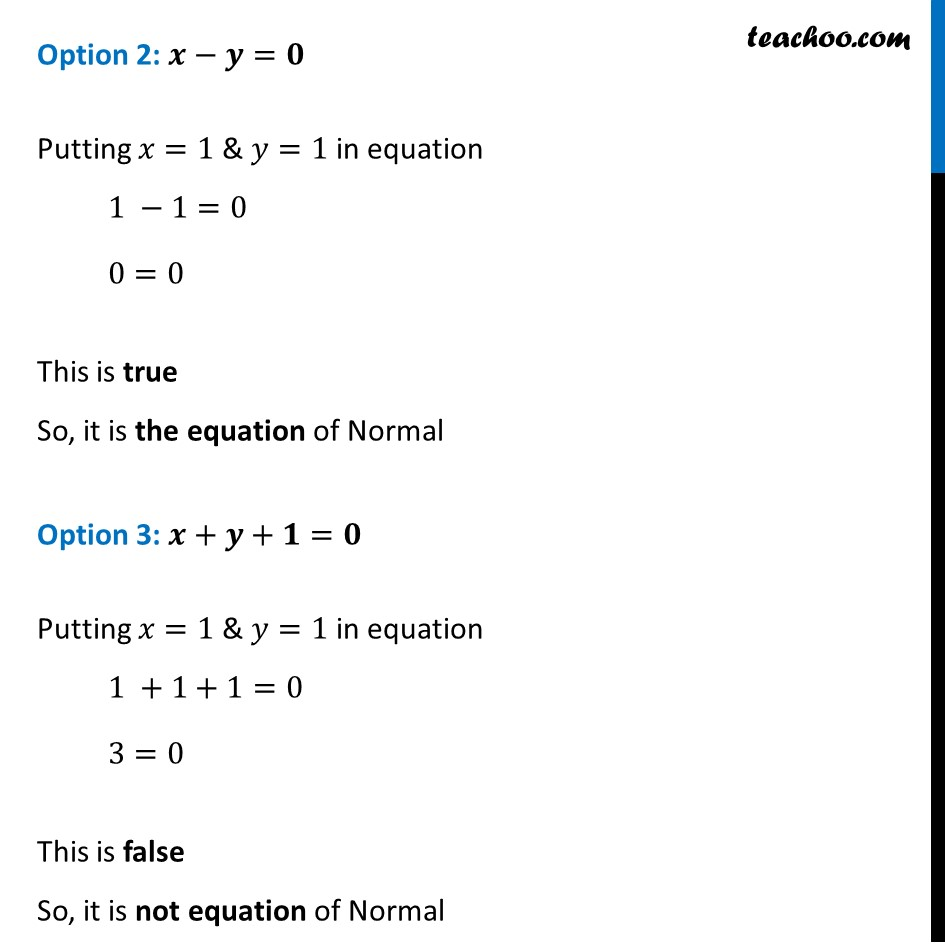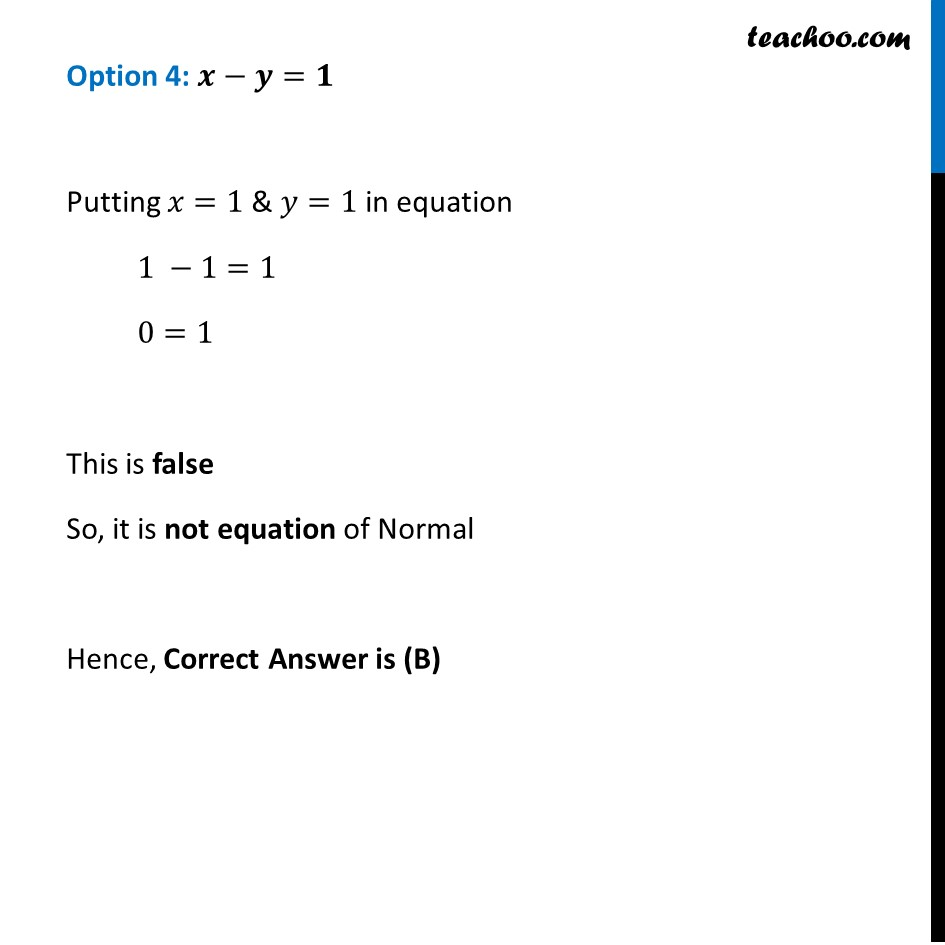Finding equation of tangent/normal when point and curve is given

Chapter 6 Class 12 Application of Derivatives
Concept wiseLearn in your speed, with individual attention - Teachoo Maths 1-on-1 Class

### Transcript

Misc 22 The normal at the point (1,1) on the curve 2𝑦+𝑥^2=3 is (𝐴) 𝑥 + 𝑦 = 0 (𝐵) 𝑥 – 𝑦 = 0 (𝐶) 𝑥 + 𝑦 +1 = 0 (𝐷) 𝑥 – 𝑦 = 1Since (1, 1) lies on normal, it will satisfy its equation Option 1: 𝒙+𝒚=𝟎 Putting 𝑥=1 & 𝑦=1 in equation 1 +1=0 2=0 This is false So, it is not equation of Normal Since (1, 1) lies on normal, it will satisfy its equation Option 1: 𝒙+𝒚=𝟎 Putting 𝑥=1 & 𝑦=1 in equation 1 +1=0 2=0 This is false So, it is not equation of Normal Option 4: 𝒙−𝒚=𝟏 Putting 𝑥=1 & 𝑦=1 in equation 1 −1=1 0=1 This is false So, it is not equation of Normal Hence, Correct Answer is (B)